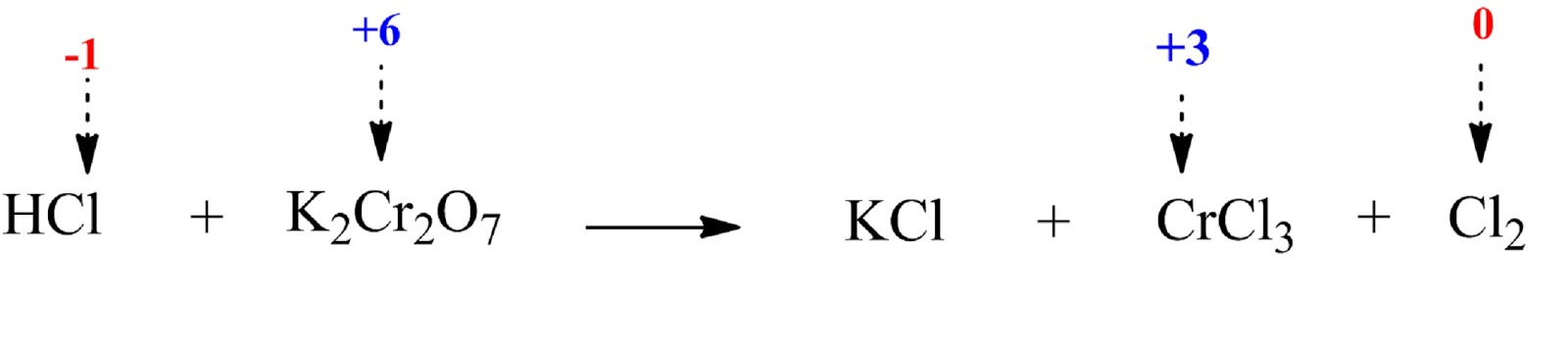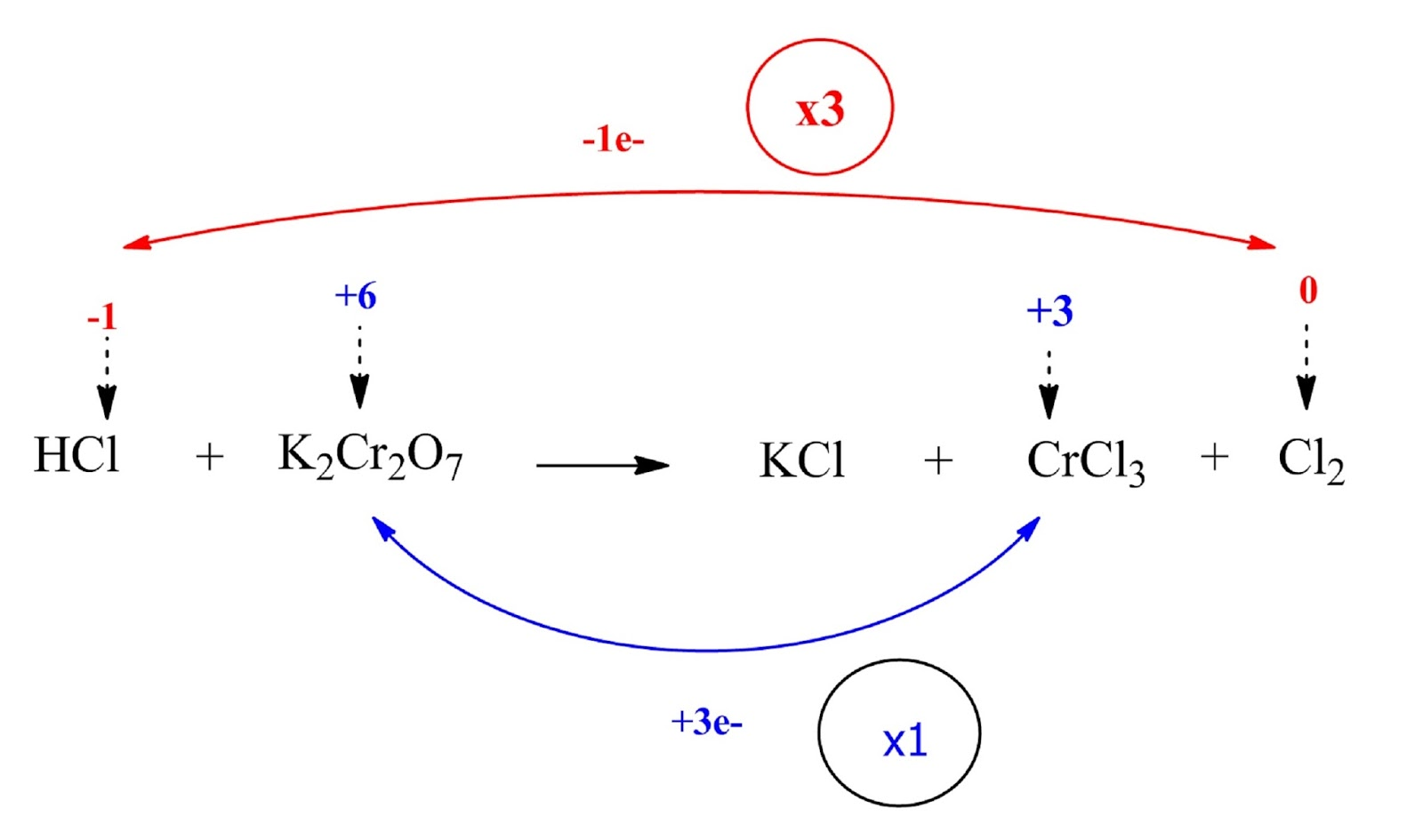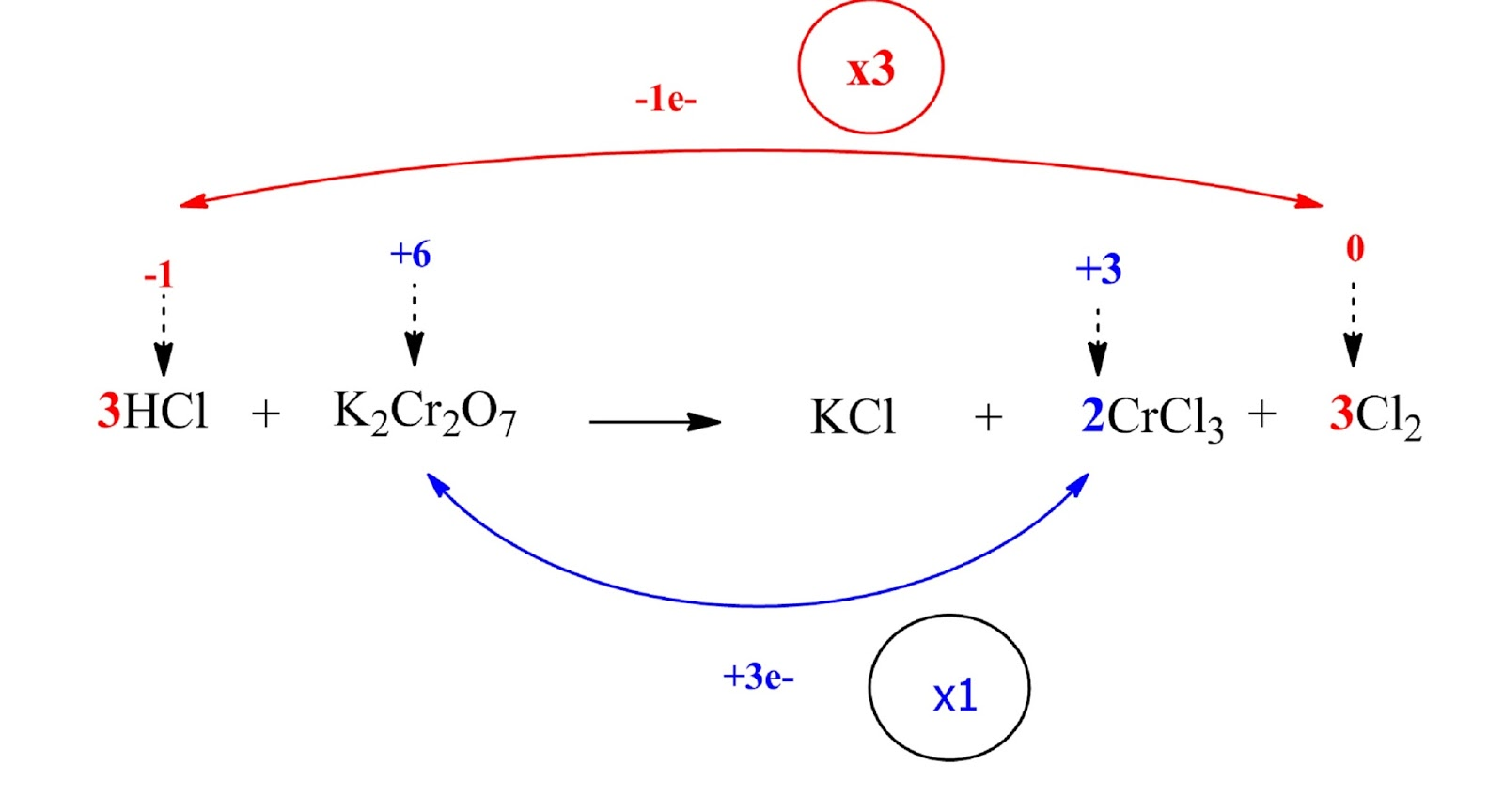Balancing Oxidation - Reduction reactions by the Oxidation Number Method | Chemistry Net

# Balancing Oxidation - Reduction reactions by the Oxidation Number Method

Balancing Oxidation / Reduction Reactions

# Balancing Oxidation - Reduction reactions by the Oxidation Number

In many important chemical reactions, electrons are transferred from atom to atom. Such reactions are called either oxidation – reduction reactions or redox reactions. Many important chemical reactions involve oxidation and reduction. In a previous post entitled “Oxidation – Reduction (Redox) Reactions – Balancing Redox Reactions” several examples of redox reactions were given and the concept of oxidation number was discussed. Solved examples for the determination of oxidation numbers to atoms in  chemical compounds were given in the post “Oxidation Numbers: Assigning oxidation numbers to atoms in a chemical compound – Examples”.

A number of different techniques have traditionally been taught in beginning chemistry students for balancing chemical reactions. These methods are the following:

• Inspection: Most students of chemistry are taught to balance simple chemical reactions by “inspection”. Each student develops his or her own personal method based on practice. However, it becomes insufficient when relatively complex redox reactions have to be balanced.
• Oxidation Number Method: This is another approach for balancing redox reactions. Oxidation numbers are assigned to atoms oxidized and reduced. By applying the general rule that the change in oxidation must be equal to the change in reduction, coefficients for these species are obtained, after which those of the remaining reactants and products.
• Half-Reaction Method: The net reaction is divided into two half-reactions, one for oxidation and the other for reduction. These half reactions are balanced separately, then combined to form a balanced reaction.
• Algebraic Method: The unbalanced chemical equation is used to define a system of linear equations, which can then be solved to yield the stoichiometric coefficients.

The oxidation number method is going to be presented in this post. The oxidation number method uses the fact that the amount of oxidation must equal the amount of reduction in the total chemical reaction. The basic steps are the following:

Step 1:   Write the correct molecular formula for each reactant and product

Step 2: Identify the elements that change oxidation number during the reaction. Write the oxidation numbers of these atoms above the appropriate chemical symbols on both sides of the equation.

Step 3:  Calculate the increase or decrease in the oxidation number per atom and for the entire molecule / ion in which it occurs. If these are not equal then multiply by suitable number so that these become equal.

Step 4:  Balance the other metal ions that do not change oxidation number

Step 5:  Add H+ (in acidic solutions) or OH- ions (in alkaline solutions) to the reaction on the appropriate side so that the total ionic charges of reactants and products are equal.

Step 6:  Make the numbers of hydrogen atoms in the expression on the two sides equal by adding H2O molecules to the reactants or products. Similarily, balance the O atoms.

Let us apply the above in the following example:

Example I.1

Balance the following reaction:

ΗCl    +    K2Cr2O7    →     KCl  +  CrCl3   +   Cl2

Step 1: Write the correct molecular formula for each reactant and product.

The correct molecular formulas are given in this case

Step 2: Identify the elements that change oxidation number during the reaction. Write the oxidation numbers of these atoms above the appropriate chemical symbols on both sides of the equation.The Cl ion is oxidized (the oxidation number increases from -1 to 0) while the Cr ion is reduced (the oxidation number decreases from +6 to +3).

Step 3: Calculate the increase or decrease in the oxidation number per atom and for the entire molecule / ion in which it occurs. If these are not equal then multiply by suitable number so that these become equal.HCl and Cl2 are multiplied by 3 (coefficient 3) while K2Cr2O7 and Cl2 by 1 (coefficient 1).Step 4:  Balance the ions that do not change oxidation number

The K atoms in our case (their oxidation number remains constant)Step 5:  Add H+ (in acidic solutions) or OH- ions (in alkaline solutions) to the reaction on the appropriate side so that the total ionic charges of reactants and products are equal.

The reaction is in acidic solution (HCl). HCl must be added on the reactants side to balance the 14 Cl atoms on the product’s side. Therefore the coefficient of HCl is going to be 14.Step 6:  Make the numbers of hydrogen atoms in the expression on the two sides equal by adding H2O molecules to the reactants or products. Similarily, balance the O atoms.

Since there are 14 H’s on the left-hand side we have to add 7H2O on the right-hand side to balance them. The reaction is now properly balanced.Relevant Posts

References

1. D. Harvey,  “Modern Analytical Chemistry”, McGraw-Hill Companies Inc., 2000
2. R.D. Brown, “Introduction to Chemical Analysis”, McGraw-Hill Companies Inc., 1982
3. S. S. Zumdahl, “Chemical Principles”, 6th Edition, Houghton Mifflin Company (2009)

Key Terms

oxidation, reduction, redox, oxidation number, balancing redox reactions,